Available In Stocks

Every Learning Objective for Fractions, Decimals & Percentages is taught by an experienced Year 6 teacher and SATs marker. Worksheets and answers included.

###### Description

All of the Fraction,s, Decimals & Percentages “Learning Objectives” from the Year 6 National Curriculum are covered in this course.

You will find video tutorials, which will guide your child through each skill, giving them time to pause the video, practice skills and learn everything they need to know to answer SATs questions at the end of the school year.

Every “Learning Objective” has it’s own video tutorial and a set of worksheets relevant to that skill. There is also a set of past SATs questions from previous years that show how each particular “Learning Objective” has been presented in the SATs previously.

Using this course, your child will ensure that they can learn every “Learning Objective” that has been set out by the  Year 6 National Curriculum from an experienced Year 6 teacher and SATs marker. They will be able to learn skills using the appropriate methods and learn test tips too.

This course is designed so that your child can work completely independently. Simply download the course onto your chosen device and they are set to go! Click on the video icon in each worksheet to access the video tutorial. All they need is a laptop, tablet or phone to view the tutorial but you can also cast the videos to your smart TV if you wanted to view the tutorials on a larger screen. Print off the worksheets to work alongside the tutorial or alternatively, view the questions on your device and use paper to write down any answers.

Contents

• use common factors to simplify fractions; use common multiples to express fractions in the same denomination
• compare and order fractions, including fractions >1
• add and subtract fractions with different denominators and mixed numbers, using the concept of equivalent fractions
• multiply simple pairs of proper fractions, writing the answer in its simplest form [for example,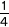×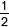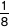]
• divide proper fractions by whole numbers [for example,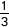÷ 2 =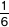]
• associate a fraction with division and calculate decimal fraction equivalents [for example, 0.375] for a simple fraction [for example,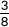]
• identify the value of each digit in numbers given to 3 decimal places and multiply and divide numbers by 10, 100 and 1,000 giving answers up to 3 decimal places
• multiply one-digit numbers with up to 2 decimal places by whole numbers
• use written division methods in cases where the answer has up to 2 decimal places
• solve problems which require answers to be rounded to specified degrees of accuracy
• recall and use equivalences between simple fractions, decimals and percentages, including in different contexts

Other available Year 6 titles:

## Reviews

There are no reviews yet.